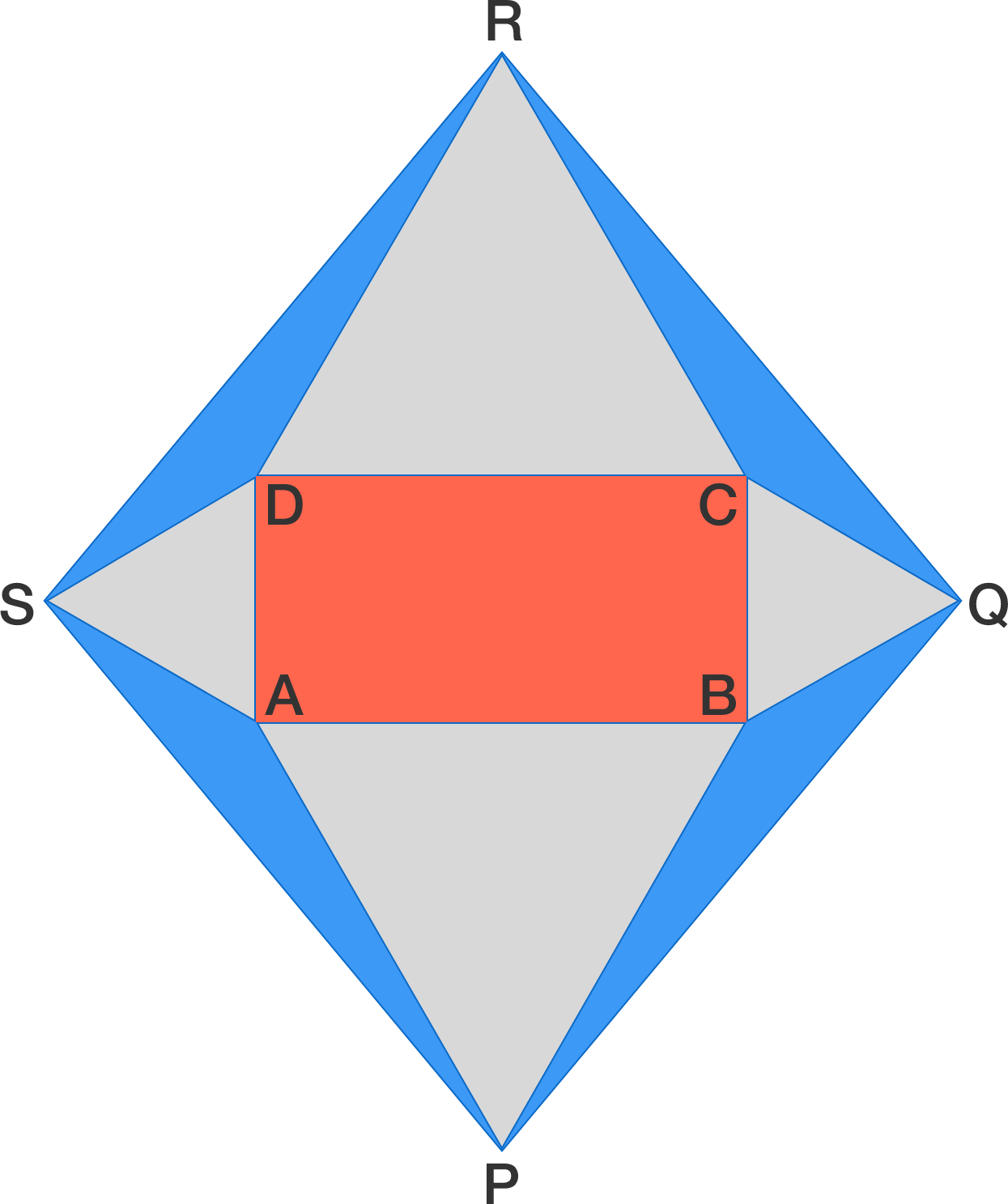# A geometry puzzle with several solutions

Geometry Level 2In the diagram above, $ABCD$ is a rectangle and triangles $ABP, BCQ, CDR, DAS$ are all equilateral. Which has a larger area, the red rectangle or the sum of the 4 blue triangles?

×